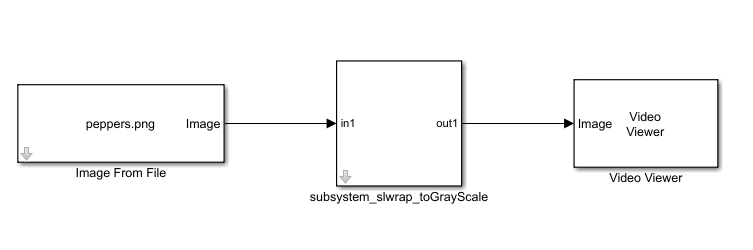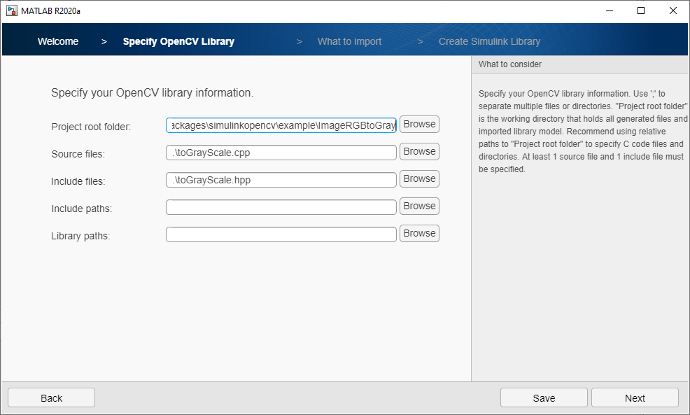Using OpenCV with MATLAB and Simulink

Using OpenCV with MATLAB and Simulink

OpenCV integrates with MATLAB® and Simulink® for collaborative development, simulation, testing, and implementation of image processing and computer vision-based systems. Through interfaces using the OpenCV C++ API, MATLAB and Simulink support integration with OpenCV.

By integrating OpenCV with MATLAB and Simulink, you can:

• Use and explore current research algorithms, whether they are implemented in MATLAB or Simulink or using OpenCV code
• Use OpenCV code with data access, image acquisition, visualization, system simulation, and testing capabilities in MATLAB and Simulink
• Use MATLAB and Simulink to explore, analyze, and debug designs that use OpenCV code
• Deploy to embedded hardware system designs that use MATLAB code, Simulink models, and OpenCV code together

MATLAB OpenCV Interface

The OpenCV interface to MATLAB makes it easy to bring single functions and entire OpenCV-based C++ projects into MATLAB using MEX. This support package requires Computer Vision Toolbox. The OpenCV interface provides:

• Prebuilt OpenCV binaries that eliminate the need to compile and build OpenCV
• Build script to create OpenCV based MEX-files
• Data type conversions between MATLAB and OpenCV
• Examples to help you get started with common workflows such as feature detection and extraction, image processing, and motion estimation

You can get started using this quick command-line example:

% After installing support package
>> mexOpenCV detectORBFeatures.cpp
% Then call this function in MATLAB just like any other MATLAB command
>> keypoints = detectORBFeatures(im);

Get started with the Computer Vision Toolbox OpenCV Interface in MATLAB documentation, which helps you download the support package, understand and use the syntax, and explore examples.

The OpenCV interface to Simulink enables you to import OpenCV code into Simulink. This support package requires Computer Vision Toolbox. The Simulink OpenCV interface enables you to:

• Import OpenCV code into a Simulink block using OpenCV Importer UI
• Generate C++ code for the Simulink model integrated with OpenCV code
• Access examples that show how to use the OpenCV Importer for designing different vision related examples

Get started with this simple example that uses a Simulink model to convert video input in RGB to grayscale using an OpenCV function in the subsystem subsystem_slwrap_toGrayScale.

The OpenCV Importer app enables you to import an OpenCV function that performs the RGB to grayscale conversion and create a Simulink library containing the equivalent block for the function. You can then integrate this block into your Simulink model.Using the subsystem subsystem_slwrap_toGrayScale that was created using the OpenCV Importer to convert an RGB input image to a grayscale output image.Specifying file locations for the OpenCV library.

Get started with the Computer Vision Toolbox OpenCV Interface in Simulink documentation, which helps you download the support package, explore and use the OpenCV Importer tool, and explore examples.

Computer Vision with MATLAB

MATLAB and Computer Vision Toolbox™ offer functionality not available in OpenCV. The toolbox provides algorithms for object detection, image recognition, and 3D lidar processing. Interactive apps such as the Camera Calibration App and Image Labeling App can save significant time when developing image algorithms.

MATLAB has new capabilities in deep learning for computer vision including access to the latest deep learning models, and training acceleration using multiple GPUs, the cloud, or clusters. You can convert your models to CUDA code with GPU Coder™. Generated CUDA code runs models up to 7x faster than TensorFlow.

Calling MATLAB from C++ and Python Applications

Interact with MATLAB functions and data types from other programming languages through MATLAB Engine:

Software Reference

See also: object detection, image recognition, object recognition, stereo vision, feature extraction, point cloud